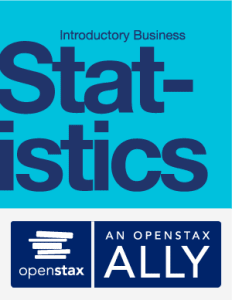# Statistics (OpenStax)

Statistics introduces learners to concepts in statistics and is geared toward students majoring in fields other than math or engineering. This course assumes some knowledge of intermediate algebra and focuses on application over theory. It also focuses on the interpretation of statistical results, especially in real world settings. Statistics includes a pre-assessment and assessment, interactive exercises, flashcards, and games that appeal to a variety of learning styles. Case studies give students opportunities to use critical thinking skills and apply their knowledge to real-world ethical scenarios.MindEdge has enhanced OpenStax courses with interactive games, video commentary, adaptive learning segments, additional practice questions, and a robust question database.

## Module 1: Sampling and Data

• Recognize and differentiate between key terms used in statistics
• Apply various types of sampling methods to data collection
• Create and interpret frequency tables

## Module 2: Descriptive Statistics

• Display data graphically and interpret graphs: stemplots, histograms, and box plots
• Recognize, describe, and calculate the measures of location of data: quartiles and percentiles
• Recognize, describe, and calculate the measures of the center of data: mean, median, and mode
• Recognize, describe, and calculate the measures of the spread of data: variance, standard deviation, and range

## Module 3: Probability

• Understand and use the terminology of probability
• Determine whether two events are mutually exclusive and whether two events are independent
• Calculate probabilities using the Addition Rules and Multiplication Rules
• Construct and interpret Contingency Tables
• Construct and interpret Venn Diagrams
• Construct and interpret Tree Diagrams

## Module 4: Discrete Random Variables

• Recognize and understand discrete probability distribution functions, in general
• Calculate and interpret expected values
• Recognize the binomial probability distribution and apply it appropriately
• Recognize the Poisson probability distribution and apply it appropriately
• Recognize the geometric probability distribution and apply it appropriately
• Recognize the hypergeometric probability distribution and apply it appropriately
• Classify discrete word problems by their distributions

## Module 5: Continuous Random Variables

• Recognize and understand continuous probability density functions in general
• Recognize the uniform probability distribution and apply it appropriately
• Recognize the exponential probability distribution and apply it appropriately

## Module 6: The Normal Distribution

• Recognize the normal probability distribution and apply it appropriately
• Recognize the standard normal probability distribution and apply it appropriately
• Compare normal probabilities by converting to the standard normal distribution

## Module 7: The Central Limit Theorem

• Recognize central limit theorem problems
• Classify continuous word problems by their distributions
• Apply and interpret the central limit theorem for means
• Apply and interpret the central limit theorem for sums

## Module 8: Confidence Intervals

• Calculate and interpret confidence intervals for estimating a population mean and a population proportion
• Interpret the Student’s t probability distribution as the sample size changes
• Discriminate between problems applying the normal and the Student’s t distributions
• Calculate the sample size required to estimate a population mean and a population proportion given a desired confidence level and margin of error

## Module 9: Hypothesis Testing with One Sample

• Differentiate between Type I and Type II Errors
• Describe hypothesis testing in general and in practice
• Conduct and interpret hypothesis tests for a single population mean, population standard deviation known
• Conduct and interpret hypothesis tests for a single population mean, population standard deviation unknown
• Conduct and interpret hypothesis tests for a single population proportion

## Module 10: Hypothesis Testing with Two Samples

• Classify hypothesis tests by type
• Conduct and interpret hypothesis tests for two population means, population standard deviations known
• Conduct and interpret hypothesis tests for two population means, population standard deviations unknown
• Conduct and interpret hypothesis tests for two population proportions
• Conduct and interpret hypothesis tests for matched or paired samples

## Module 11: The Chi-Square Distribution

• Interpret the chi-square probability distribution as the sample size changes
• Conduct and interpret chi-square goodness-of-fit hypothesis tests
• Conduct and interpret chi-square test of independence hypothesis tests
• Conduct and interpret chi-square homogeneity hypothesis tests
• Conduct and interpret chi-square single variance hypothesis tests

## Module 12: Linear Regression and Correlation

• Discuss basic ideas of linear regression and correlation
• Create and interpret a line of best fit
• Calculate and interpret the correlation coefficient
• Calculate and interpret outliers

## Module 13: F Distribution and One-Way ANOVA

• Interpret the F probability distribution as the number of groups and the sample size change
• Discuss two uses for the F distribution: one-way ANOVA and the test of two variances
• Conduct and interpret one-way ANOVA
• Conduct and interpret hypothesis tests of two variances